Courses

Test: FIR System Structures - 1

15 Questions MCQ Test Digital Signal Processing | Test: FIR System Structures - 1

Description
Attempt Test: FIR System Structures - 1 | 15 questions in 10 minutes | Mock test for Electrical Engineering (EE) preparation | Free important questions MCQ to study Digital Signal Processing for Electrical Engineering (EE) Exam | Download free PDF with solutions
QUESTION: 1

In general, an FIR system is described by the difference equation: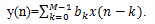Solution: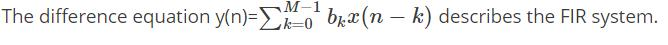QUESTION: 2

Which of the following is an method for implementing an FIR system?

Solution:
• There are several structures for implementing an FIR system, beginning with the simplest structure, called the direct form.
• There are several other methods like cascade form realization, frequency sampling realization and lattice realization which are used for implementing and FIR system.
QUESTION: 3

The direct form realization is often called a transversal or tapped-delay-line filter.

Solution:

The structure of the direct form realization, resembles a tapped delay line or a transversal system.

QUESTION: 4

What is the general system function of an FIR system?

Solution: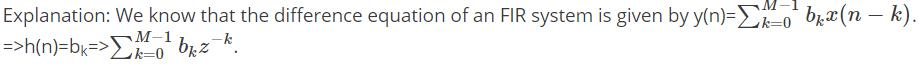QUESTION: 5

How many memory locations are used for storage of the output point of a sequence of length M in direct form realization?

Solution:
• The direct form realization follows immediately from the non-recursive difference equation given by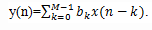• We observe that this structure requires M-1 memory locations for storing the M-1 previous inputs.
QUESTION: 6

What is the condition of M, if the structure according to the direct form is as follows?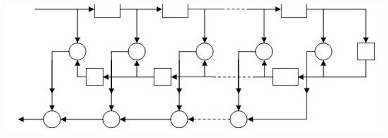Solution:
• When the FIR system has linear phase, the unit sample response of the system satisfies either the symmetry or asymmetry condition, h(n)= ±h(M-1-n)
• For such a system the number of multiplications is reduced from M to M/2 for M even and to (M-1)/2 for M odd. Thus for the structure given in the question, M is odd.
QUESTION: 7

By combining two pairs of poles to form a fourth order filter section, by what factor we have reduced the number of multiplications?

Solution:
• We have to do 3 multiplications for every second order equation.
• So, we have to do 6 multiplications if we combine two second order equations and we have to perform 3 multiplications by directly calculating the fourth order equation.
• Thus the number of multiplications are reduced by a factor of 50%.
QUESTION: 8

The desired frequency response is specified at a set of equally spaced frequencies defined by the equation:

Solution:
• To derive the frequency sampling structure, we specify the desired frequency response at a set of equally spaced frequencies, namely:
• ωk=2π / M(k+α) ,k=0,1…(M-1)/2 for M odd
• k=0,1….(M/2)-1 for M even α = 0 or 0.5.
QUESTION: 9

The realization of FIR filter by frequency sampling realization can be viewed as cascade of how many filters?

Solution:
• In frequency sampling realization, the system function H(z) is characterized by the set of frequency samples {H(k+ α)} instead of {h(n)}.
• We view this FIR filter realization as a cascade of two filters. One is an all-zero or a comb filter and the other consists of parallel bank of single pole filters with resonant frequencies.
QUESTION: 10

What is the system function of all-zero filter or comb filter?

Solution: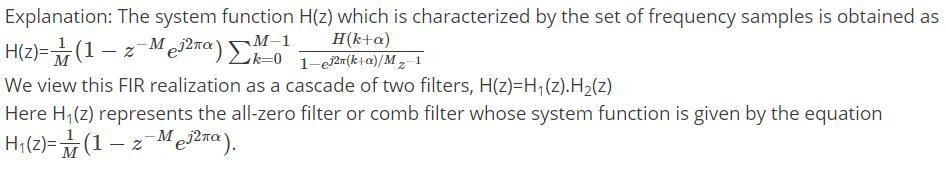QUESTION: 11

The zeros of the system function of comb filter are located:

Solution: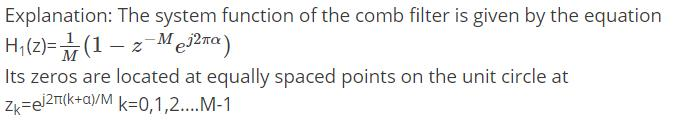QUESTION: 12

What is the system function of the second filter other than comb filter in the realization of FIR filter?

Solution: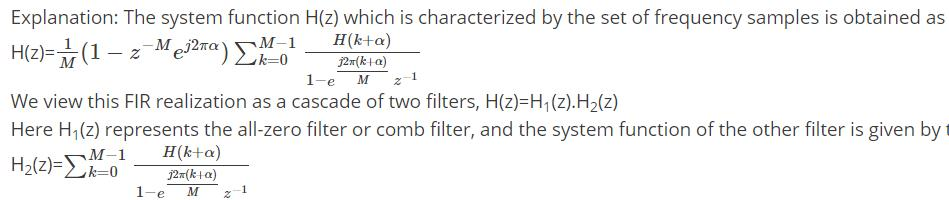QUESTION: 13

Where does the poles of the system function of the second filter locate?

Solution: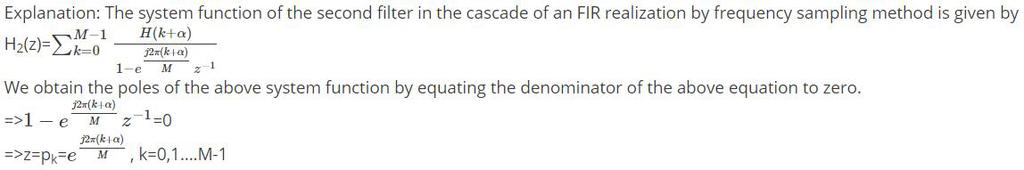QUESTION: 14

When the desired frequency response characteristic of the FIR filter is narrowband, most of the gain parameters {H(k+α)} are zero.

Solution:

Explanation: When the desired frequency response characteristic of the FIR filter is narrowband, most of the gain parameters {H(k+α)} are zero. Consequently, the corresponding resonant filters can be eliminated and only the filters with nonzero gains need be retained.

QUESTION: 15

Which of the following filters have a cascade realization as shown below?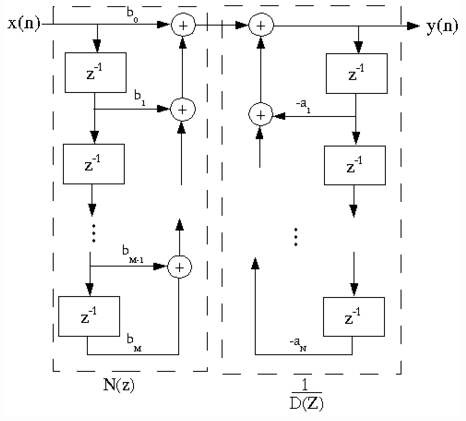Solution:

Explanation: The system function of the FIR filter according to the frequency sampling realization is given by the equation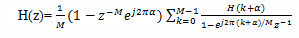The above system function can be represented in the cascade form as shown in the above block diagram.Use Code STAYHOME200 and get INR 200 additional OFF Use Coupon Code

Track your progress, build streaks, highlight & save important lessons and more!

Similar ContentRelated tests